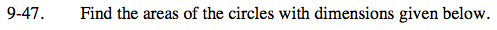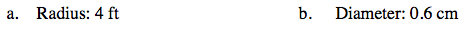Home > CC2MN > Chapter cc29 > Lesson cc29.1.3 > Problem9-47

9-47.
1. Find the areas of the circles with dimensions given below. Homework Help ✎

1. Radius: 4 ft

2. Diameter: 0.6 cmA = πr2

A = πr2

$\textit{r}=\frac{\text{diameter}}{2}=\frac{0.6\text{ cm}}{2}=0.3\text{ cm}$

A = π(0.3 cm)2

Approximately 0.2826 sq cm